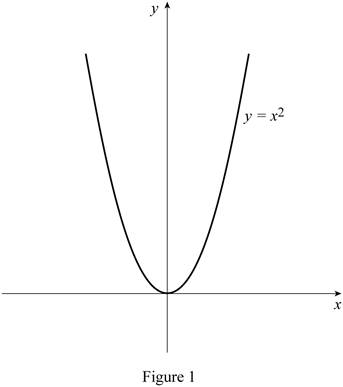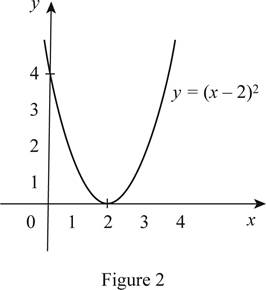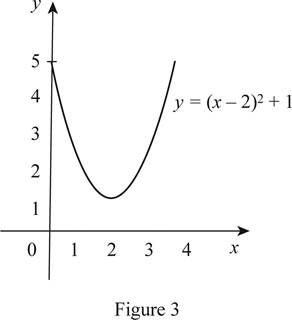# The function y = x 2 by hand without plotting any points and then graph of the function y = x 2 − 4 x + 5 .### Single Variable Calculus: Concepts...

4th Edition
James Stewart
Publisher: Cengage Learning
ISBN: 9781337687805### Single Variable Calculus: Concepts...

4th Edition
James Stewart
Publisher: Cengage Learning
ISBN: 9781337687805

#### Solutions

Chapter 1.3, Problem 15E
To determine

## To sketch: The function y=x2 by hand without plotting any points and then graph of the function y=x2−4x+5 .

Expert Solution

### Explanation of Solution

It is given that y=x24x+5 .

Express the given function as follows.

y=x24x+5=x24x+4+1=(x2)2+1

Thus, draw the graph of y=(x2)2+1 .

The standard graph of the function y=x2 is roughly drawn as shown below in Figure 1.Then, draw the graph of y=(x2)2 , shift the graph y=x2 by 2 units to the right. Thus, the graph of y=(x2)2 is shown below in Figure 2.From Figure 2, it is observed that Figure 1 is shifted 2 units to the right.

To draw the graph of y=(x2)2+1 , shift the graph y=(x2)2 by 1 unit upward. Thus, the graph of y=(x2)2+1 is shown below in Figure 3.Observe that Figure 3is obtainedfrom Figure 1 in such a way that it is shifted 2 units to the right and then shifted 1 unit upward.

### Have a homework question?

Subscribe to bartleby learn! Ask subject matter experts 30 homework questions each month. Plus, you’ll have access to millions of step-by-step textbook answers!